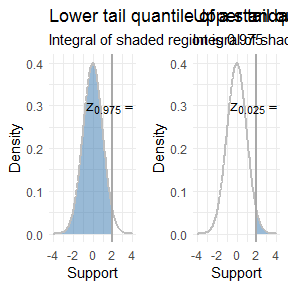# Z confidence interval for a mean

The normal distribution also comes up frequently when calculating confidence intervals for sample means. Let’s calculate a 88 percent confidence interval for the same data from vignette("one-sample-z-test"), which was:

$3, 7, 11, 0, 7, 0, 4, 5, 6, 2$

Again we will assume that $$\sigma = 2$$. If you would like to see how to calculate a confidence interval for the mean of a single sample when you don’t know the population standard deviation $$\sigma$$, see vignette("one-sample-t-confidence-interval").

Recall that a confidence interval for the mean based off the normal distribution is valid when:

1. The data comes from a normal distribution.
2. We have lots of data. How much? Many textbooks use 30 data points as a rule of thumb.

In the Z-test example we verified that the sample seems to come from a normal distribution using a quantile-quantile plot (QQ-plot).

The formula for a confidence interval with confidence coefficient $$1 - \alpha$$ (in our case this is 0.88) is then:

$\left( \bar x + z_{\alpha / 2} \cdot \frac{\sigma}{\sqrt{n}}, \bar x + z_{1 - \alpha / 2} \cdot \frac{\sigma}{\sqrt{n}} \right)$

Where $$z_\alpha$$ stands for the alpha-ith quantile of a standard normal distribution. To get a quantile we need to take the inverse of the c.d.f. so you may also see $$z_\alpha$$ written as $$\Phi^{-1}(\alpha)$$, where $$\Phi$$ is the c.d.f. of the standard normal. Since the standard normal distribution is symmetric around zero, this is exactly equivalent to

$\left( \bar x - z_{1 - \alpha / 2} \cdot \frac{\sigma}{\sqrt{n}}, \bar x + z_{1 - \alpha / 2} \cdot \frac{\sigma}{\sqrt{n}} \right)$

which may look slightly more familiar. Having trouble seeing what the difference is? Look at the subscript for $$z$$. Let’s go ahead and calculate this out in R. Since our confidence coefficient is 0.88 (corresponding to an 88 percent confidence interval) we have:

$0.88 = 1 - \alpha$

so that $$\alpha = 0.12$$. Now we can get started.

library(distributions3)

x <- c(3, 7, 11, 0, 7, 0, 4, 5, 6, 2)
n <- length(x)

# make a standard normal r.v.
Z <- Normal(0, 1)

# first approach
mean(x) + quantile(Z, 0.12 / 2) * 2 / sqrt(n)
#>  3.516675
mean(x) + quantile(Z, 1 - 0.12 / 2) * 2 / sqrt(n)
#>  5.483325

So our confidence interval using the first set of equations is (3.52, 5.48). Now we use the second set of equations:

# second approach
mean(x) - quantile(Z, 1 - 0.12 / 2) * 2 / sqrt(n)
#>  3.516675
mean(x) + quantile(Z, 1 - 0.12 / 2) * 2 / sqrt(n)
#>  5.483325

We get the same thing! Just like we expected.

There’s one last thing we need to address. You may not have seen either of the formulas for a Z-confidence interval that I wrote. You may have seen the formulas:

$\left( \bar x - z_{\alpha / 2} \cdot \frac{\sigma}{\sqrt{n}}, \bar x + z_{\alpha / 2} \cdot \frac{\sigma}{\sqrt{n}} \right)$

If this is you, you have my condolences, and your instructor probably hates you. Ask that they use lower quantile notation rather than upper quantile notation.

This looks almost like the second approach, except using $$z_{\alpha / 2}$$ instead of $$z_{1 - \alpha / 2}$$. What this comes down to is whether or not $$z_{\alpha / 2}$$ represents a lower quantile or an upper quantile. For a lower quantile, you look at the p.d.f. and start integrating from negative infinity, stop when the integral equals $$\alpha$$, and that take value to be the quantile. This is the only sane way to do things, although it requires being slightly more verbose so it can be inconvenient at times. Thus the upper quantile, in which case you do the same integration but start from positive infinity.

The difference is easiest to understand with a picture:Note that distributions always returns a lower quantile from the quantile() function.

If you are truly unfortunate, your instructor may use $$z_{\alpha / 2}$$ to mean lower tail quantiles at times and upper tail quantiles at other times. If this is the case, only god can help you.